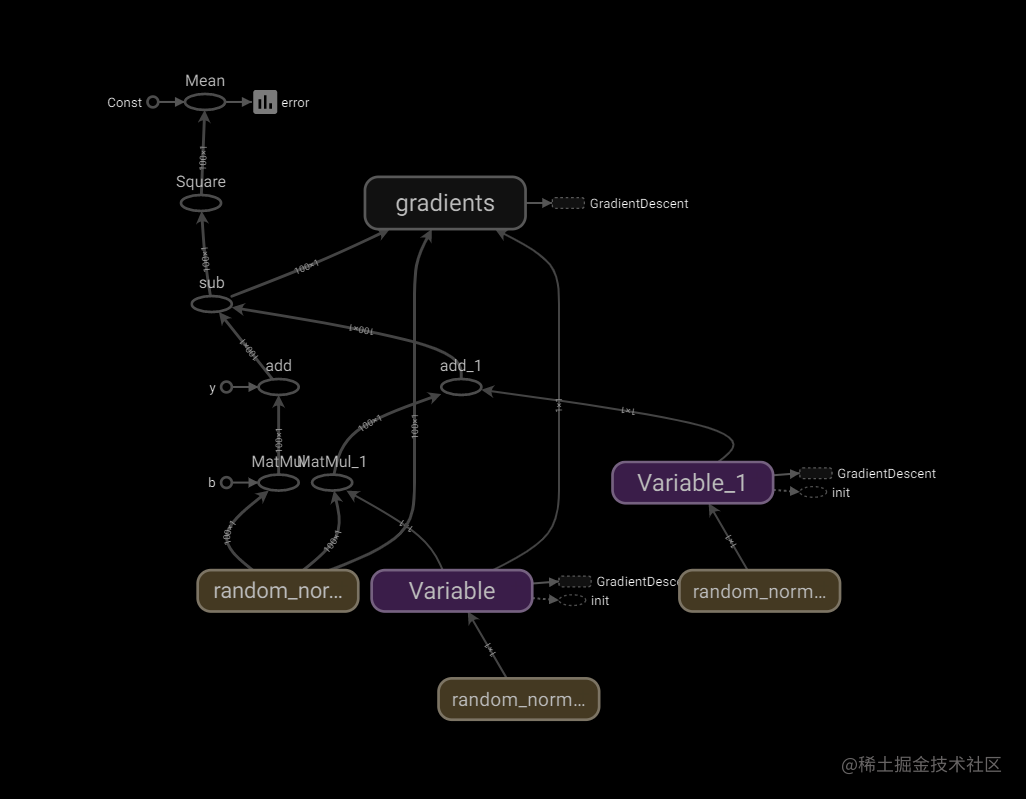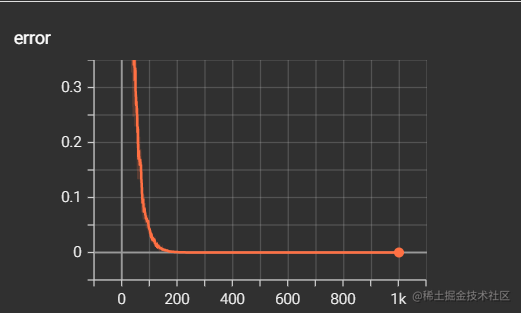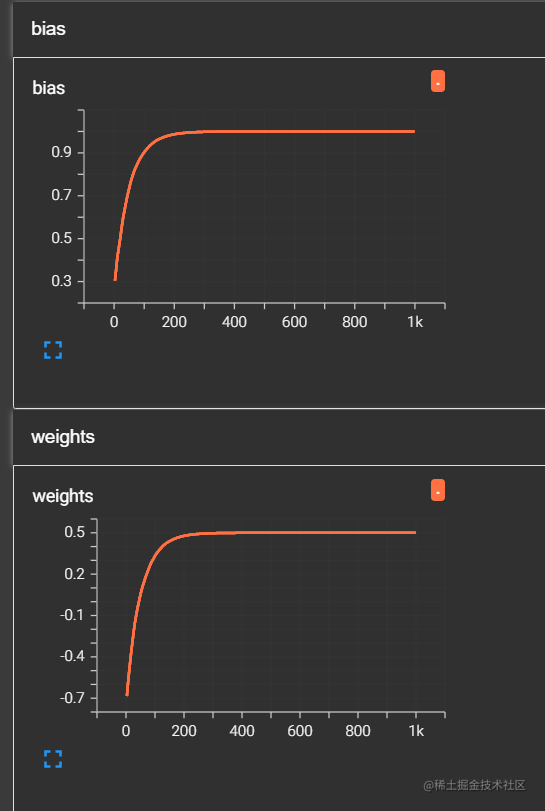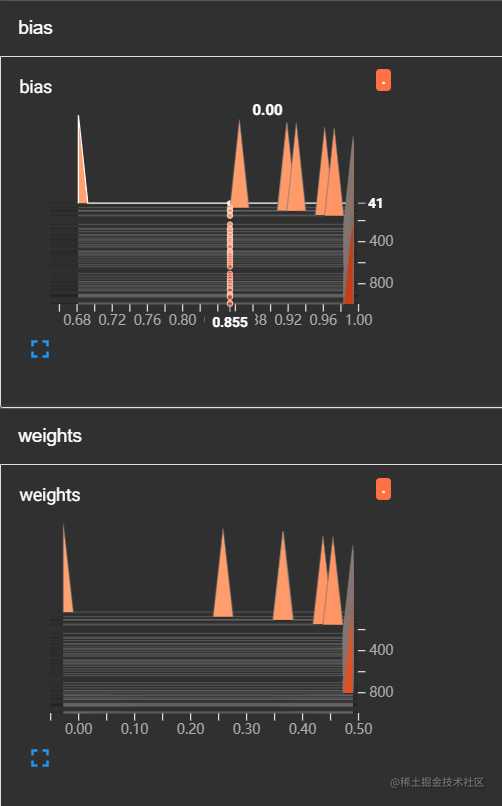# 【深度学习】TensorFlow线性回归案例演示（3）

highlight: a11y-dark theme: nico

# 3. 代码演示

### 步骤1：导入所需库

```ipython import tensorflow as tf import tensorflow.compat.v1 as tf tf.disable_v2_behavior()```

### 步骤2：线性回归并收集变量

• 我们在`./File/linear`目录下创建了事件文件，用来保存变量中间结果
• 需要在训练时的每一次循环后将变量写入事件
• 本案例演示中，主要用到收集变量与变量合并写入事件，最终得到可视化结果
• 主要流程为：

1. 创建事件
2. 收集变量
3. 合并变量
4. 写入变量 ```ipython def linear_regression(): """ 实现线性回归 """

# 1.1 准备数据

X = tf.random_normal(shape=[100,1]) y_true = tf.matmul(X, [[0.5]]) + 1 # matmul矩阵运算

# 用变量来定义

weights = tf.Variable(initial_value=tf.random_normal(shape=[1,1])) # 需要给一个初始设置：正态分布随机初始值；一行一列 bias = tf.Variable(initial_value=tf.random_normal(shape=[1,1])) y_predict = tf.matmul(X, weights) + bias

# 1.3 损失函数

error = tf.reduce_mean(tf.square(y_predict-y_true))

# 2) 收集变量

tf.summary.scalar("error", error) tf.summary.histogram("weights", weights) tf.summary.histogram("bias", bias)

# 3) 合并变量

merged = tf.summary.merge_all()

# 2. 初始化变量

init = tf.global_variables_initializer()

# 3. 开启会话

with tf.Session() as sess: sess.run(init)

``````# 1) 创建事件文件
file_writer = tf.summary.FileWriter("./File/linear", graph=sess.graph)

# 查看初始化模型参数的值
print("训练前模型参数为：权重%f, 偏置%f, 损失%f" %(weights.eval(), bias.eval(), error.eval()))

# 4. 开始训练
for i in range(1000):
sess.run(optimizer)
print("第%d次训练后模型参数为：权重%f, 偏置%f, 损失%f" %(i+1, weights.eval(), bias.eval(),error.eval()))

# 运行合并变量操作
summary = sess.run(merged)
# 将每次迭代后的变量写入事件文件
``````

return None

linear_regression() ``` 经过1000轮训练得到的结果如下图所示：### 步骤3：TensorBoard可视化File文件中是刚才保存变量的事件，需要Tensorboard将其打开查看。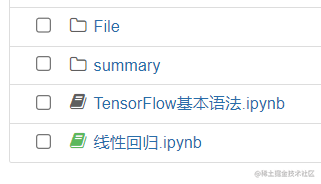`cd 文件保存上级目录path`

`tensorboard --logdir=./File/linear/`